# 2 Water potential is a concept that helps

• Slides: 20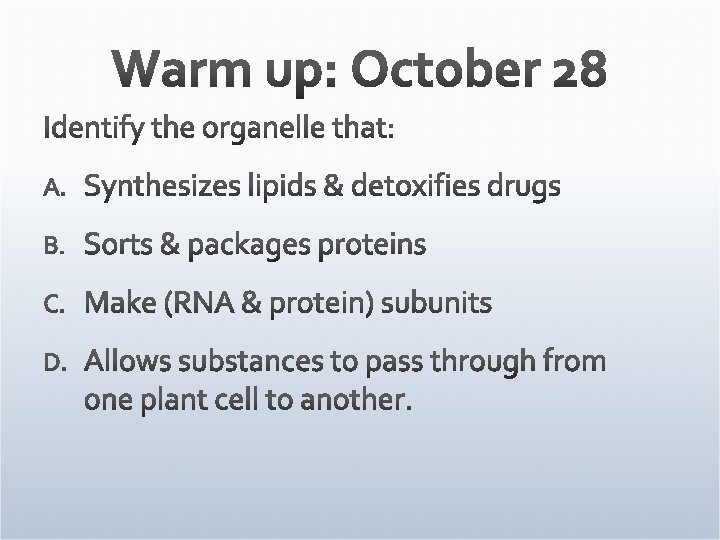2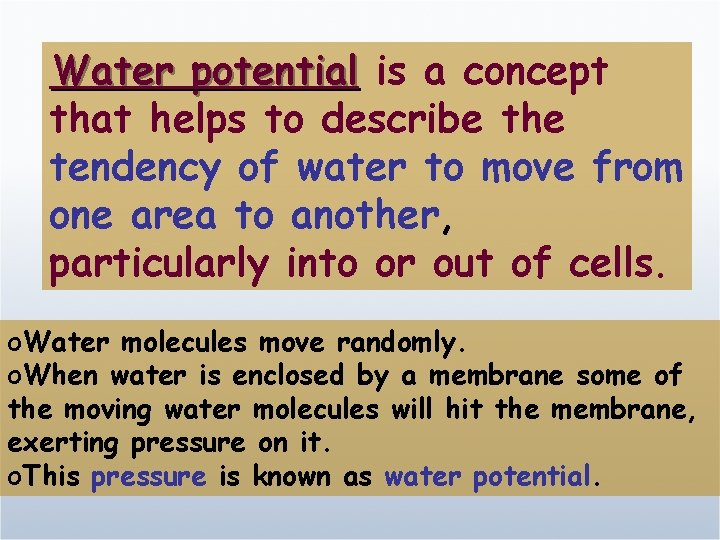Water potential is a concept that helps to describe the tendency of water to move from one area to another, particularly into or out of cells. o. Water molecules move randomly. o. When water is enclosed by a membrane some of the moving water molecules will hit the membrane, exerting pressure on it. o. This pressure is known as water potential.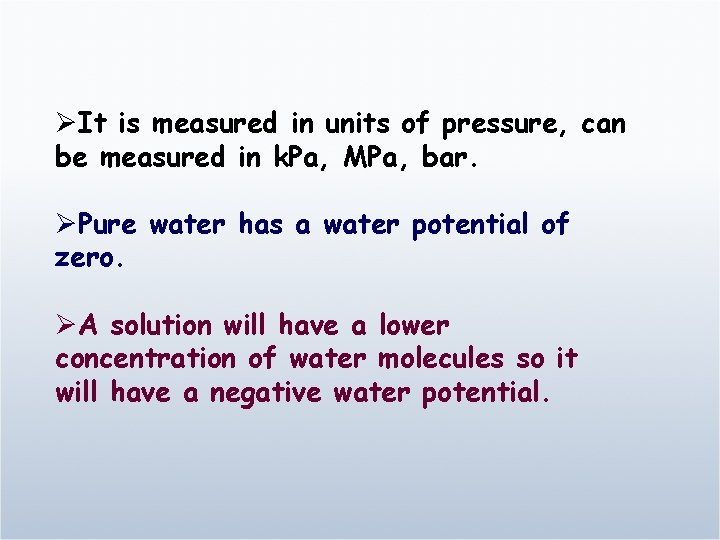ØIt is measured in units of pressure, can be measured in k. Pa, MPa, bar. ØPure water has a water potential of zero. ØA solution will have a lower concentration of water molecules so it will have a negative water potential.water movement water potential l Solute concentration pressure Water potential determines the rate and direction of osmosis.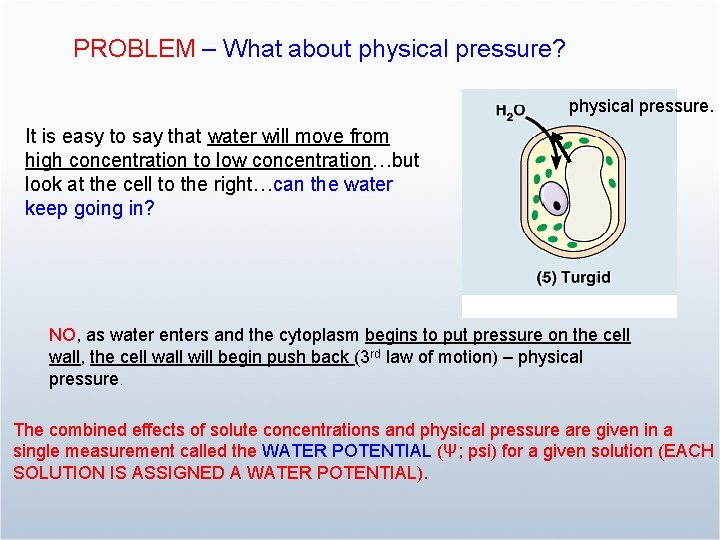PROBLEM – What about physical pressure? physical pressure. It is easy to say that water will move from high concentration to low concentration…but look at the cell to the right…can the water keep going in? NO, as water enters and the cytoplasm begins to put pressure on the cell wall, the cell wall will begin push back (3 rd law of motion) – physical pressure. The combined effects of solute concentrations and physical pressure are given in a single measurement called the WATER POTENTIAL (Ψ; psi) for a given solution (EACH SOLUTION IS ASSIGNED A WATER POTENTIAL).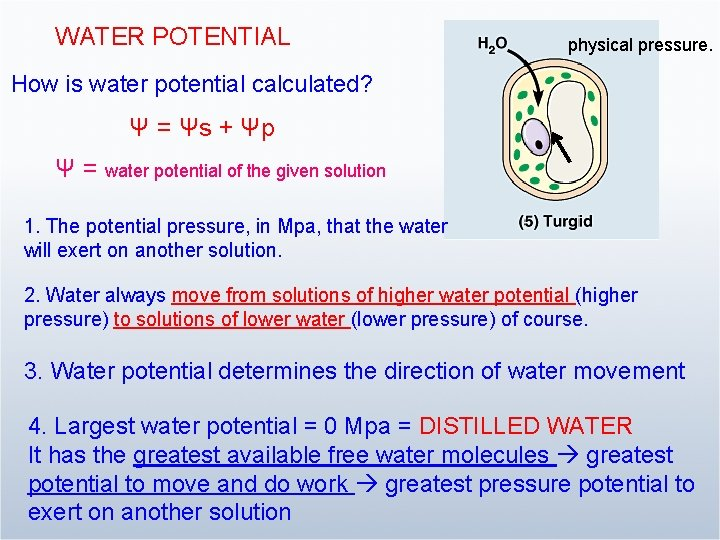WATER POTENTIAL physical pressure. How is water potential calculated? Ψ = Ψs + Ψp Ψ = water potential of the given solution 1. The potential pressure, in Mpa, that the water will exert on another solution. 2. Water always move from solutions of higher water potential (higher pressure) to solutions of lower water (lower pressure) of course. 3. Water potential determines the direction of water movement 4. Largest water potential = 0 Mpa = DISTILLED WATER It has the greatest available free water molecules greatest potential to move and do work greatest pressure potential to exert on another solution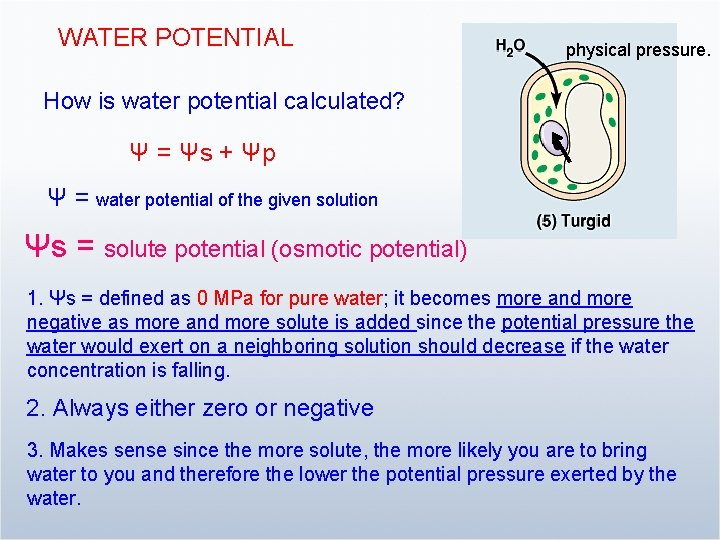WATER POTENTIAL physical pressure. How is water potential calculated? Ψ = Ψs + Ψp Ψ = water potential of the given solution Ψs = solute potential (osmotic potential) 1. Ψs = defined as 0 MPa for pure water; it becomes more and more negative as more and more solute is added since the potential pressure the water would exert on a neighboring solution should decrease if the water concentration is falling. 2. Always either zero or negative 3. Makes sense since the more solute, the more likely you are to bring water to you and therefore the lower the potential pressure exerted by the water.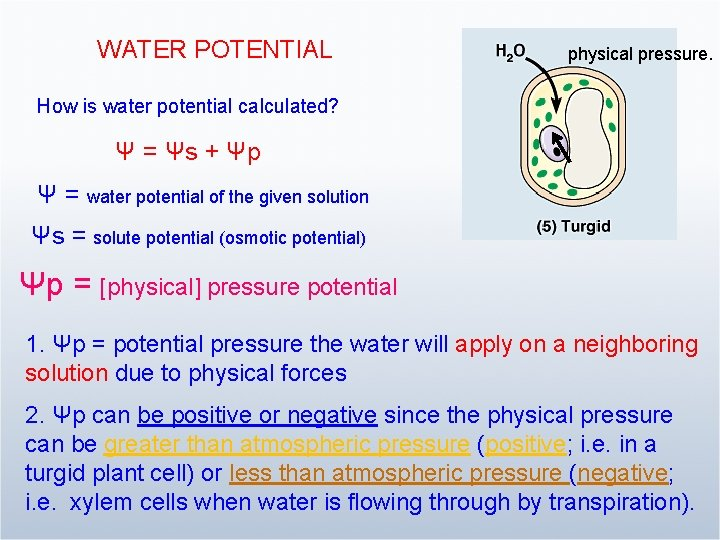WATER POTENTIAL physical pressure. How is water potential calculated? Ψ = Ψs + Ψp Ψ = water potential of the given solution Ψs = solute potential (osmotic potential) Ψp = [physical] pressure potential 1. Ψp = potential pressure the water will apply on a neighboring solution due to physical forces 2. Ψp can be positive or negative since the physical pressure can be greater than atmospheric pressure (positive; i. e. in a turgid plant cell) or less than atmospheric pressure (negative; i. e. xylem cells when water is flowing through by transpiration).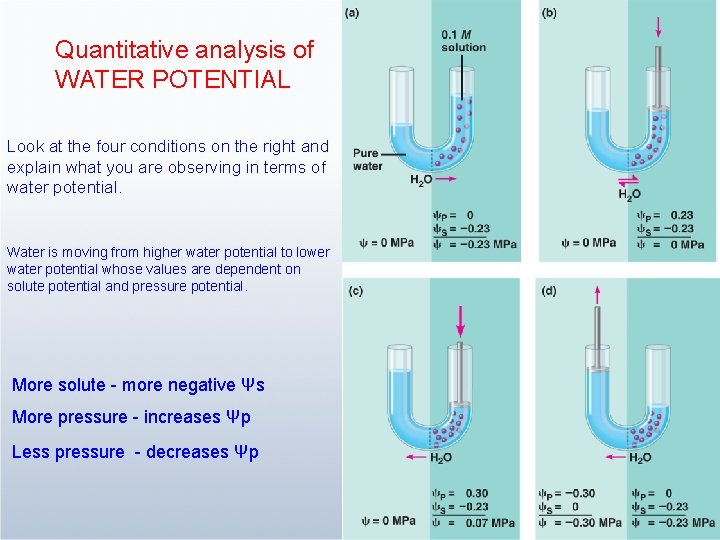Quantitative analysis of WATER POTENTIAL Look at the four conditions on the right and explain what you are observing in terms of water potential. Water is moving from higher water potential to lower water potential whose values are dependent on solute potential and pressure potential. More solute - more negative Ψs More pressure - increases Ψp Less pressure - decreases ΨpQuantitative analysis of WATER POTENTIAL Hypertonic Hyp 0 tonic Look at the conditions on the right and left: explain what you are observing in terms of water potential. The cell is at equilibrium when the cell wall pushes back with the equivalent pressure potential of 0. 7 MPa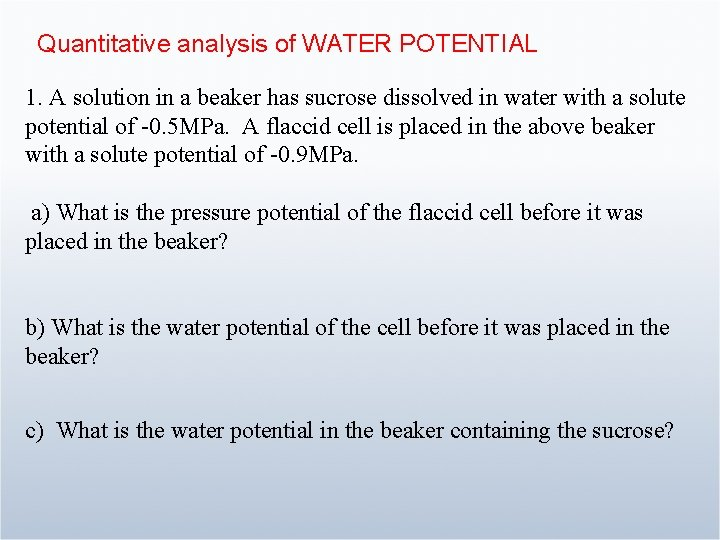Quantitative analysis of WATER POTENTIAL 1. A solution in a beaker has sucrose dissolved in water with a solute potential of -0. 5 MPa. A flaccid cell is placed in the above beaker with a solute potential of -0. 9 MPa. a) What is the pressure potential of the flaccid cell before it was placed in the beaker? b) What is the water potential of the cell before it was placed in the beaker? c) What is the water potential in the beaker containing the sucrose?Quantitative analysis of WATER POTENTIAL d) How will the water move? e) What is the pressure potential of the plant cell when it is in equilibrium with the sucrose solution outside? Also, what is its final water potential when it is in equilibrium? f) Is the cell now turgid/flaccid/plasmolysed? g) Is the cell hypotonic or hypertonic with respect to the outside prior to equilibrium?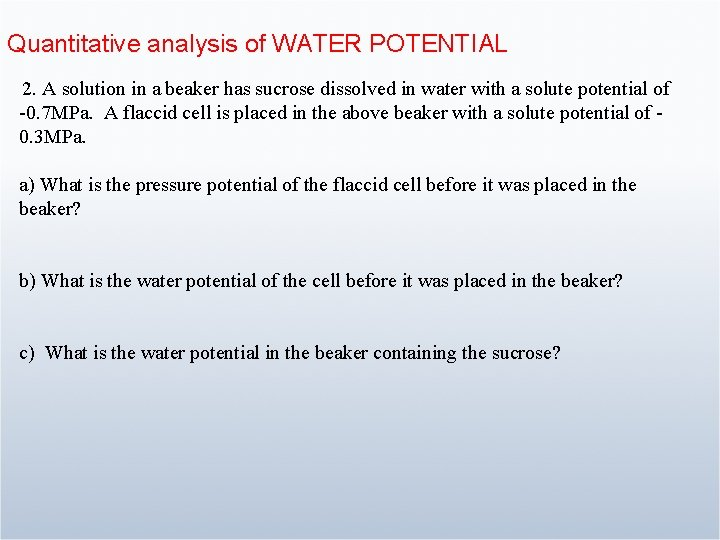Quantitative analysis of WATER POTENTIAL 2. A solution in a beaker has sucrose dissolved in water with a solute potential of -0. 7 MPa. A flaccid cell is placed in the above beaker with a solute potential of 0. 3 MPa. a) What is the pressure potential of the flaccid cell before it was placed in the beaker? b) What is the water potential of the cell before it was placed in the beaker? c) What is the water potential in the beaker containing the sucrose?Quantitative analysis of WATER POTENTIAL d) How will the water move? e) What is the pressure potential of the plant cell when it is in equilibrium with the sucrose solution outside? Think carefully – does the plant cell wall change shape? f) Also, what is the cell’s final water potential when it is in equilibrium? g) Is the cell now turgid/flaccid/plasmolysed?Quantitative analysis of WATER POTENTIAL h) What is the cell’s solute potential when it is in equilibrium? I) Is the cell hypotonic or hypertonic with respect to the outside prior to equilibrium? J) If it is hypo/hyper (choose one) tonic – this means that its water potential is higher/lower (choose one) than the outside.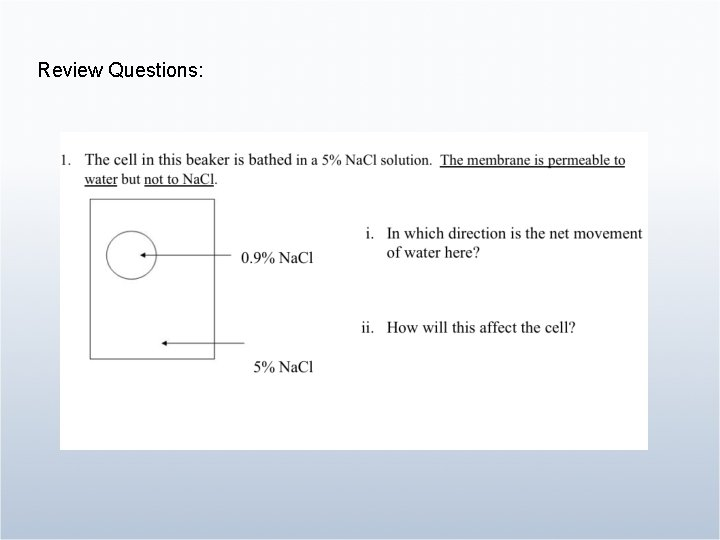Review Questions: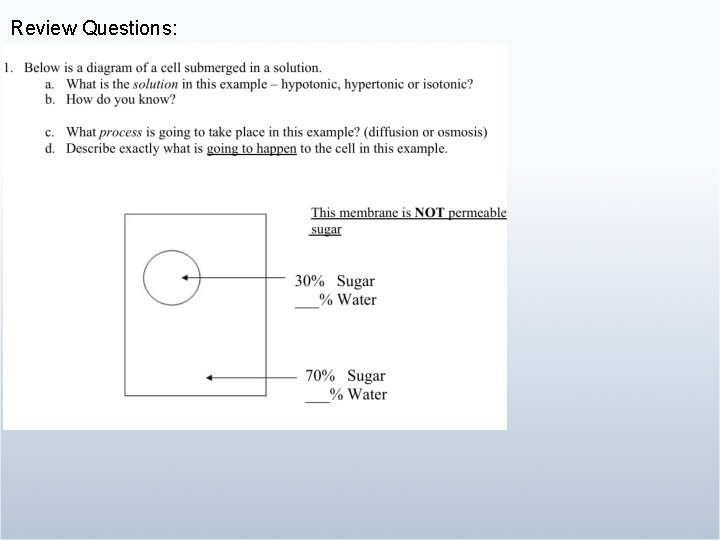Review Questions: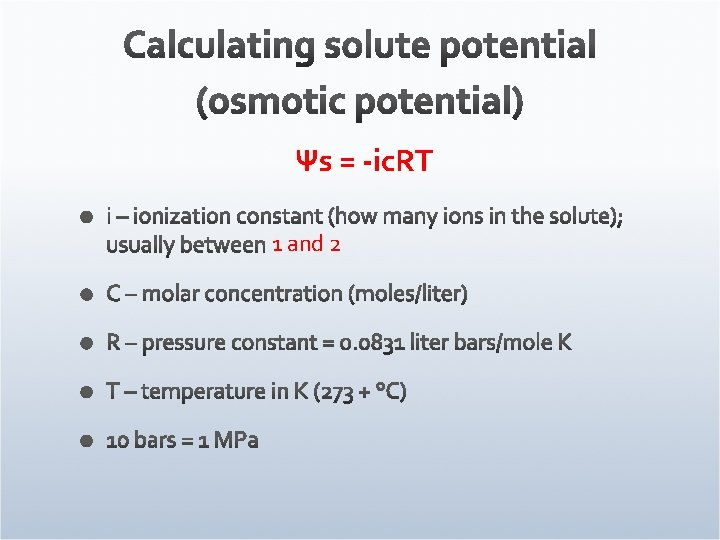Ψs = -ic. RT 1 and 2Ans: . 8 bars Ans: -4. 9 bars